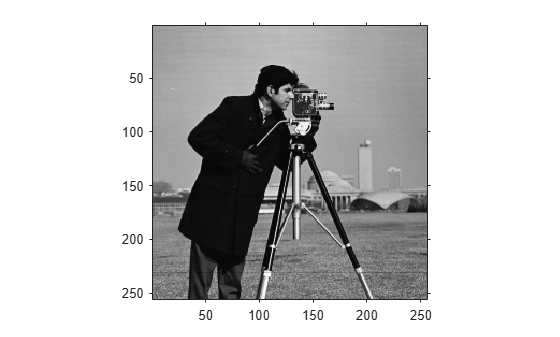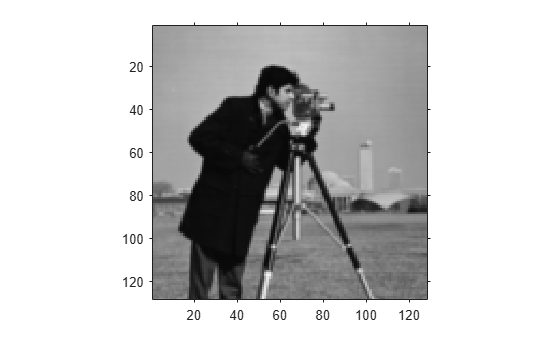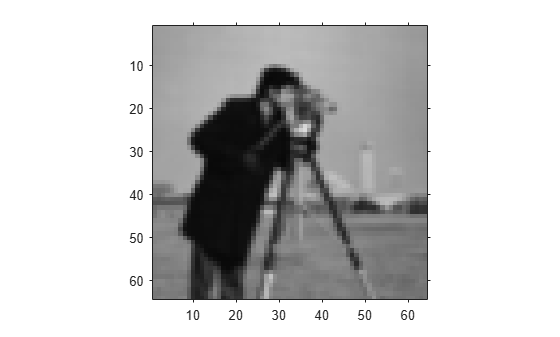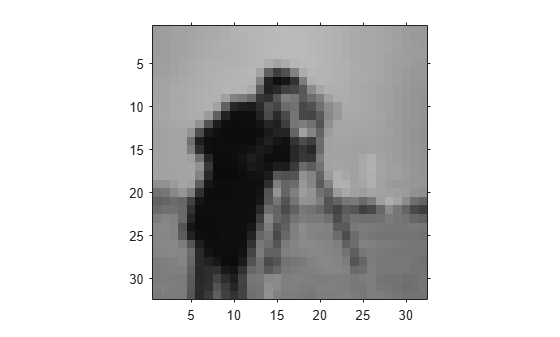# impyramid

Image pyramid reduction and expansion

## Syntax

``B = impyramid(A,direction)``

## Description

example

````B = impyramid(A,direction)` computes a Gaussian pyramid reduction or expansion of `A` by one level. `direction` determines whether `impyramid` performs a reduction or an expansion.```

## Examples

collapse all

`I = imread('cameraman.tif');`

Perform a series of reductions. The first call reduces the original image. The other calls to `impyramid` use the previously reduced image.

```I1 = impyramid(I, 'reduce'); I2 = impyramid(I1, 'reduce'); I3 = impyramid(I2, 'reduce');```

View the original image and the reduced versions.

`figure, imshow(I)``figure, imshow(I1)``figure, imshow(I2)``figure, imshow(I3)`## Input Arguments

collapse all

Image to reduced or expanded, specified as a numeric or logical array.

Data Types: `single` | `double` | `int8` | `int16` | `int32` | `uint8` | `uint16` | `uint32` | `logical`

Reduction or expansion, specified as one of the following values:

ValueDescription
`"reduce"`Return an image, smaller than the original image.
`"expand"`Return an image that is larger than the original image.

Data Types: `char` | `string`

## Output Arguments

collapse all

Reduced or expanded image, returned as a numeric or logical array, the same class as `A`.

## Algorithms

If `A` is m-by-n and `direction` is `"reduce"`, the size of `B` is `ceil(M/2)`-by-`ceil(N/2)`. If `direction` is `"expand"`, the size of `B` is `(2*M-1)`-by-`(2*N-1)`.

Reduction and expansion take place only in the first two dimensions. For example, if `A` is 100-by-100-by-3 and `direction` is `"reduce"`, then `B` is 50-by-50-by-3.

`impyramid` uses the kernel specified on page 533 of the Burt and Adelson paper:

$w=\left[\frac{1}{4}-\frac{a}{2},\frac{1}{4},a,\frac{1}{4},\frac{1}{4}-\frac{a}{2}\right]$, where . The parameter a is set to `0.375` so that the equivalent weighting function is close to a Gaussian shape. In addition, the weights can be readily applied using fixed-point arithmetic.

 Burt and Adelson, "The Laplacian Pyramid as a Compact Image Code," IEEE Transactions on Communications, Vol. COM-31, no. 4, April 1983, pp. 532-540.

 Burt, "Fast Filter Transforms for Image Processing," Computer Graphics and Image Processing, Vol. 16, 1981, pp. 20-51